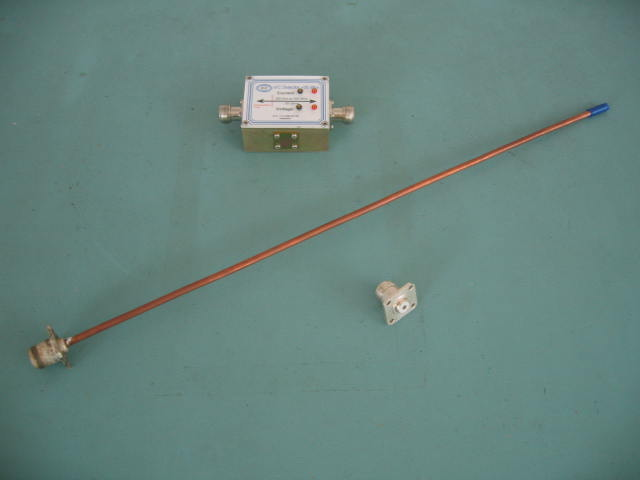# Antenna Current Distribution Page 4

We now investigate if current is flowing to the very end of an antenna by making electrical length measurements using current. The antenna is operated as a half wave end fed dipole (with 180° of phase shift in one direction) in order to minimise any reactive antenna components which would adversely affect the accuracy of the measurement. There is the added advantage that a half wave antenna is a very poor match to 50 Ohms and therefore reflects most of the power into it, giving readily observable nulls in the response of a vector difference current measurement. The antenna length and cable length are carefully selected to enable the antenna to operate at the point where they are both half wavelengths long.

The lengths of the cable and antenna are first selected to operate within both the frequency range of the test equipment and the physical space available for the antenna, which in the example given meant that the antenna was as short as possible without exceeding the upper frequency range of the test equipment used (in this case, 300 MHz for the highest frequency of the Current/Voltage Detector). A coaxial cable of the correct mechanical length is built using the Equation 1.1 which uses the cables velocity factor to find the required mechanical length at a half wave.Equation 1.1

Where c is the speed of light in m/s (3 x 108)

Vf is the velocity factor

f is the frequency in Hz

An antenna is made from a length of copper wire, rod or tube which is soldered to a suitable connector. The length of the antenna being selected using Equation 1.1 and a velocity factor of 1. A second, identical connector is modified as a cable extension by removing the connection part of the centre pin, see figure 10. Also shown is a Voltage/Current Detector made by us.

Figure 10The test equipment is first connected as per figure 11 in order to find the frequency for the current null at 180° of phase shift in one direction (half wave) of the test cable with the modified connector. The DMM is connected to the current output of the Voltage/Current Detector. By measuring first a quarter wave with the cable and then a half wave for the cable and antenna, the measurement frequency is kept almost constant and therefore, any frequency response measurement error in the test equipment is minimised. The modified connector is used in order obtain the electrical length of the measurement set up from the Voltage/Current Detector to the very base of the antenna. Those familiar with vector network analyser measurements will recognise this as establishing the measurement reference plane at the very base of the antenna. The Broadband Amplifier and Voltage and Current Detector designed by Chemandy Electronics can be used for this measurement and a description of the functioning of the Current/Voltage detector can be found at Appendix C

Figure 11The Signal Generator is set to a low frequency which is then gradually increased until a null is reached on the DMM at approximately the calculated quarter wave frequency. The electrical length is then calculated from the frequency of the Signal Generator using Equation 1.2 (the frequency in this case being 279.1 MHz, giving an electrical length of 0.537 metre for a total mechanical length of 0.356 metre, which gives the correct velocity factor of 0.66 for this cable).Equation 1.2

The circuit is then re-configured as per figure 12 and the antenna length is trimmed to achieve a current null. The generator frequency can be changed during this process in order to assess how the trimming is progressing but should be returned to the same frequency as found in the cable measurement. The overall electrical length of the cable and antenna is then calculated using Equation 1.2 (which in this case is a total length of 1.076 metres). The electrical length of the antenna is then calculated by subtracting the two electrical lengths obtained (which in this case gives an antenna electrical length of 1.076 - 0.537 = 0.539 metres).

Figure 12It can be seen that the electrical length (in this case 0.539 metres) of the antenna is longer than the mechanical length of the antenna (in this case 0.444 metres). If the electrical length of the antenna was shorter than the mechanical length then it would be conclusive proof that the current does not reach the end of the antenna, but this is not the case. The velocity constant of the antenna is now calculated by dividing the mechanical length by the electrical length (in this case 0.444 / 0.539 = 0.8237) and this constant is very similar to that of a high quality coaxial cable which is in the region of 0.82, thus showing that the antenna is functioning like a lossy transmission line, in which the current does in fact reach the very end. There is no evidence to suggest that the current does not reach the end of an antenna and it has already been proven on pages 2 and 3 that a current transformer at the end of the antenna would indicate zero current, as there are two almost equal currents of virtually the same phase at this point. It is therefore assumed in the rest of this study that the current does actually reach the very end of the antenna where it is reflected towards the original input.

1  2  3  4  5  6  7   >  Pages

How Does an Antenna Work Index

Technical Article Index